# The Effect of Geometrical Parameters on Enhancing the Heat Transfer Inside a Microtube

The Effect of Geometrical Parameters on Enhancing the Heat Transfer Inside a Microtube

B. H. SalmanH. A. Mohammed A. SH. Kherbee Hamdi E. Ahmed

Department of Mechanical Engineering, University of Nevada, Las Vegas, 89154 NV, USA

Department of Thermofluids, Universiti Teknologi Malaysia, 81310 UTM Skudai, Johor Bahru, Malaysia

Mechanical Engineering Department, Universiti Tenaga Nasional, 43000 Kajang, Selangor, Malaysia

Department of Mechanical Engineering, University of Anbar, 31001 Anbar, Iraq

Corresponding Author Email:
eng.bassam2007@yahoo.com
Page:
79-84
|
DOI:
http://doi.org/10.18280/ijht.330311
N/A
|
Accepted:
N/A
|
Published:
30 September 2015
| Citation

OPEN ACCESS

Abstract:

In this paper, the effect of geometrical parameters of microtube on enhancing the heat transfer has been studied numerically. Single phase model was used to simulate the flow of sio2-ethlyen glycol nanofluids inside a microtube at different reynolds numbers ranged from 10 to 160 at constant heat flux boundary condition. The results show that the higher tube diameter and entrance size has the highest Nusselt number and lower pressure drop. Furthermore, no effect of inclination angles was found.

Keywords:

Numerical modelling, Nanofluids, Microtube, Heat transfer enhancement.

1. Introduction

In recent years, liquid suspensions containing nanoparticles gained significant attention in science and engineering. Their unique thermophysical properties, such as a drastically reduced melting temperature of the nanoparticles compared to their bulk counterpart [1, 2], allow for novel methods in the manufacturing of nano and microelectronic devices. Many researchers intended to investigate experimentally and numerically the effects of the microtube and microchannel shapes, sizes, heat transfer and the other parameters that can be influence on the performance for the microelectronic devices. However, the recent numerical and experimental researches that related to microtube have been summarized in Table1.

The purpose of this work is to provide a numerical study on the effect of geometrical parameters (entrance size, shape and inclination angle on enhancing the heat transfer at different Reynolds number ranged from 10 to 120 for different inclination angles ranged from 0° to ±90°.

2. Numerical Model

2.1 Geometry and the governing equations

Navier–Stokes and energy equations were used to describe the flow and heat transfer in the microtube. The following assumptions are adopted: (i) The nanofluid is incompressible, the flow is laminar, the radiation heat transfer effects are negligible; (ii) The nanoparticles are assumed to be spherical and single phase model is used; (iii) Constant thermophysical properties are considered for the nanofluid. The governing equations used are as follows:

Continuity equation:

$\frac{1}{R} \frac{\partial}{\partial \theta}\left(\rho_{e f f} U\right)+\frac{1}{R} \frac{\partial}{\partial R}\left(\rho_{e f f} R V\right)+\frac{\partial}{\partial Z}\left(\rho_{e f f} W\right)=0$    (1)

Momentum equation:

$\theta$ -Components

$1 \frac{\partial}{\partial \theta}\left(\rho_{e f f} U U\right)+\frac{1}{R} \frac{\partial}{\partial R}\left(\rho_{e f f} R V U\right)+\frac{\partial}{\partial Z}\left(\rho_{e f f} W U\right)+\frac{1}{R}\left(\rho_{e f f} U V\right) =-\frac{1}{R^{2}} \frac{\partial P}{\partial \theta}+\frac{1}{R^{2}} \frac{\partial}{\partial \theta}\left(\mu_{e f f} \frac{\partial U}{\partial \theta}\right)+\frac{\partial}{\partial R}\left(\frac{\mu_{e f f} }{R} \frac{\partial(R U)}{\partial R}\right)+\frac{2\mu_{e f f}}{R^{2}} \frac{\partial V}{\partial \theta}$   (2a)

R- Components

$\frac{1}{R} \frac{\partial}{\partial \theta}\left(\rho_{e f f} U V\right)+\frac{1}{R} \frac{\partial}{\partial R}\left(\rho_{e f f} R V V\right)+\frac{\partial}{\partial Z}\left(\rho_{e f f} W V\right)-\frac{1}{R}\left(\rho_{e f f} U^{2}\right) =-\frac{1}{R} \frac{\partial P}{\partial \theta}+\frac{1}{R^{2}} \frac{\partial}{\partial \theta}\left(\mu_{e f f} \frac{\partial V}{\partial \theta}\right)+\frac{\partial}{\partial R}\left(\frac{\mu_{e f f}}{R} \frac{\partial(R V)}{\partial R}\right)-\frac{2 \mu_{e f f}}{R^{2}} \frac{\partial U}{\partial \theta}$  (2b)

Z- Components

$\frac{1}{R} \frac{\partial}{\partial \theta}\left(\rho_{e f f} U W\right)+\frac{1}{R} \frac{\partial}{\partial R}\left(\rho_{e f f} R V W\right)+\frac{\partial}{\partial Z}\left(\rho_{e f f} W W\right)= =-\frac{\partial P}{\partial Z}+\frac{1}{R^{2}} \frac{\partial}{\partial \theta}\left(\mu_{e f f} \frac{\partial W}{\partial \theta}\right)+\frac{1}{R} \frac{\partial}{\partial R}\left(R \mu_{e f f} \frac{\partial W}{\partial \theta}\right)$  (2c)

Energy equation:

$\frac{1}{R} \frac{\partial}{\partial \theta}\left(\rho_{e f f} U \Theta\right)+\frac{1}{R} \frac{\partial}{\partial R}\left(\rho_{e f f} R V \Theta\right)+\frac{\partial}{\partial Z}\left(\rho_{e f f} W \Theta\right) =\frac{1}{R^{2}} \frac{\partial}{\partial \theta}\left(\frac{K e f f}{(C p)_{e f f}} \frac{\partial \Theta}{\partial \theta}\right)+\frac{\partial}{R \partial R}\left(R \frac{K e f f}{(C p)_{e f f}} \frac{\partial \Theta}{\partial R}\right)$      (3)

Where the non-dimensional variables are defined as

$R=\frac{r}{D}, \quad Z=\frac{z}{D}, \quad U=\frac{u}{u_{\infty}}, \quad V=\frac{v}{u_{\infty}}, \quad W=\frac{w}{u_{\infty}}, \quad \Theta=\frac{T-T}{T_{w}-T_{\infty}}, \quad P=\frac{p}{\rho u_{\infty}^{2}}$

The $\rho_{e f f}, \mu_{e f f}, C_{e f f}$ and $k_{e f f}$ are density, viscosity, heat capacity and thermal conductivity of nanfluid, respectively.

2.2 Boundary conditions

At the tube inlet, the inlet temperature was taken as Tin=301 K. No-slip conditions and uniform heat flux used was 50000 W/m2 to heat up the tube. Different velocities depending on the values of Reynolds number were used. At the tube outlet, the flow and heat transfer are assumed to be fully developed.

2.3 Nanofluids thermophysical properties

The thermophysical properties which are density, heat capacity, dynamic viscosity and thermal conductivity for SiO2–EG nanofluid are given in Table 2. These properties are calculated using the following equations:

Effective thermal conductivity :

$k_{e f f}=k_{\text {static}}+k_{\text {Brownian}}$   (4)

$k_{\text {static}}=k_{f}\left[\frac{\left(k_{n p}+2 k_{f}\right)-2 \phi\left(k_{f}-k_{n p}\right)}{\left(k_{n p}+2 k_{f}\right)+\phi\left(k_{f}-k_{n p}\right)}\right]$    (5)

Where $k_{n p}$ and $k_{f}$ are the thermal conductivity of the solid particles and the base fluid respectively.

Thermal conductivity due to the Brownian motion presented by  as:

$k_{\text {Brownian}}=5 \times 10^{4} \beta \phi p_{f} c_{p f} \sqrt{\frac{K T}{2 R_{n p}}} f(T, \phi)$

$f(T, \phi)=(0.028217 \varnothing+0.003917) \frac{T}{T_{0}}+(-0.030669 \varnothing-0.00391123)$     (6)

Where K is the Boltzman constant, T is the fluid temperature; T0 is the reference temperature, the $\beta$ values for SiO2 particle expressed in as follow :

$\begin{array}{llll}1.9526(100 \phi)-1.4594 & \text { for } & 1 \% \leq \varphi \leq 10 \% & \text { at } 298 K \leq T \leq 363 K\end{array}$   (7)

The effective dynamic viscosity is given as :

$\frac{\mu_{e f f}}{\mu_{f}}=\frac{1}{1-34.87\left(d_{p} / d_{f}\right)^{-0.3} \varnothing^{1.03}}$     (8)

Where $d f=\left[\frac{6 \times M}{N \times \pi \times \rho_{f 0}}\right]^{1 / 3}$    (9)

While $\mu_{e f f}$ and $\mu_{f}$ are the viscosity of nanofluid and base fluid respectively, $d_{p}$  is the nanoparticle diameter, $d_{f}$ is the base fluid equivalent diameter and  is the nanoparticle volume fraction. M is the molecular weight of the base fluid and N is the Avogadro number. $\rho_{f_{0}}$  is the mass density of the base fluid calculated at temperature T0=293 K.

The effective density is given as :

$\rho_{e f f}=(1-\varnothing) \rho_{f}+\varnothing \rho_{s}$    (10)

Where $\rho_{e f f}$ and $\rho_{f}$ are the nanofluid and base fluid densities respectively, and $\rho_{s}$  is the density of nanoparticle.

The effective specific heat is given as :

$(\mathrm{Cp})_{\mathrm{eff}}=\frac{(1-\varnothing)\left(\rho C_{p}\right)_{f}+\varnothing\left(\rho C_{p}\right)_{x}}{(1-\varnothing) \rho_{f}+\varnothing \rho_{s}}$     (11)

Where C p_{s} is the heat capacity of the solid particles and c p_{f} is the heat capacity of the base fluid.

Table 2. Thermophysical properties of nanofluids.

 Base fluids Nanoparticle Properties EG SiO2 ρ (kg/m3) 1114.4 2200 μ (Nm/s) 0.0157 - k (W/mK) 0.252 1.2 cp (kJ/kgK) 2415 703

2.4 Numerical implementation

The Finite volume approach is used to solve the continuity, momentum and energy equations along with the corresponding boundary conditions. SIMPLE algorithm is used to solve the flow field inside the MT. The diffusion term in the momentum and energy equations is approximated by second-order central difference which gives a stable solution. The second-order upwind differencing scheme is considered for the convective terms. Five different sets of the grid sizes were imposed to the geometry and simulated by calculating the Nusselt number along the MT. The five grids sizes ($8 \times 6 \times 200$ ; $10 \times 8 \times 400$ ; $12 \times 10 \times 600$ ;$14 \times 12 \times 800$ and $16 \times 14 \times 1000$ ) show no much difference in the values of Nusselt number. The grid size of $14 \times 12 \times 800$ is selected in this study as it is found to provide a more stable solution. About the code validation part is documented in the Ref. .

3. Results and Discussion

The simulations are performed for Reynolds number in the range of 10 ≤ Re ≤ 160 and SiO2 nanoparticle with pure EG as a base fluid. The nanoparticles volume fraction used was 0.04 with nanoparticles diameter 25nm. The MT used has a 100 mm length with different diameters ranged from 0.5 to 0.9 mm and different entrance sizes ranged from 0.371 to 2.5 mm. The heat flux that used to heat up the microtube was 50000 W/m2. The inclination angles from the horizontal position were 0°, ± 45°, ± 90° are used in this investigation.

Table 1. Summary of numerical and experimental studies for MT

## biao_1.png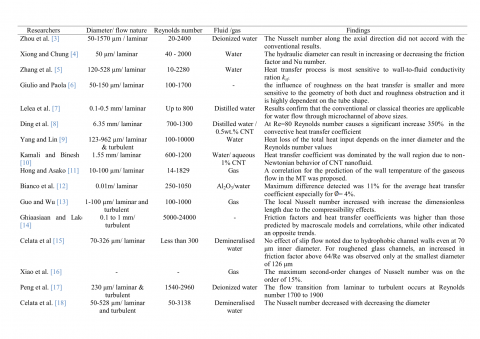## biao_2.png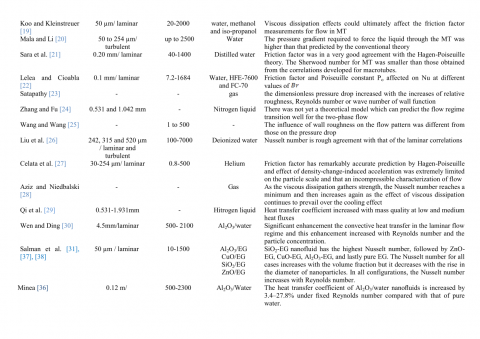3.1 Effect of tube diameter

The effect of tube geometry on, the axial and wall temperatures, axial velocity along the tube radius, Nusselt number and the pressure drop are presented in this section.

In Figure 1a and 1b temperature profile at z/L=1 along the tube radius and tube axis shows that the tube with 0.9 mm diameter has the highest temperature along the tube radius and tube wall followed by 0.7 mm, 0.5 mm respectively. This is because the velocity proportional inversely with the tube diameter. Smaller diameter increases the velocity which leads to decrease the temperature and vice versa.

## 1a.png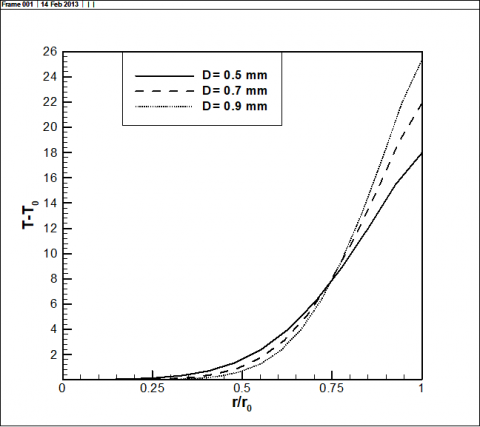(a)

## 1b.png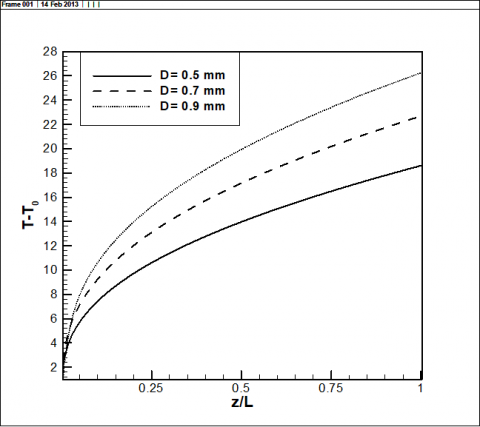(b)

Figure 1. Profiles of temperature at z/L=1for different tube diameters: (a) Temperature along the tube radius; (b) Temperature along tube axis

In Figure 2 the axial velocity along tube radius profile shows that the tube with 0.5 mm diameter has the highest

axial velocity along the tube radius at z/L=0.2 for Reynolds number Re=80 followed by 0.7 mm, then 0.9 mm. This is because the smaller tube diameter is proportional directly to the velocity.

Figure 3 shows the average Nusselt number for different Reynolds numbers. It can be obtained from this Figure that 0.9 mm has the highest Nusselt number comparing to other tubes. The larger tube diameter has highest Nusselt number. This is because for Nusselt number is proportional directly with the tube diameter; higher tube diameter leads to higher Nusselt number and vice versa. Figure 4 the pressure drop along the tube axis show that the tube with 0.5 mm diameter has the highest pressure drop followed by 0.7 mm and 0.9 mm. This is because the static pressure for the fluid is proportional directly with the velocity. Thus, 0.5 mm tube has the highest velocity which leads to increases the pressure drop.

## 2.png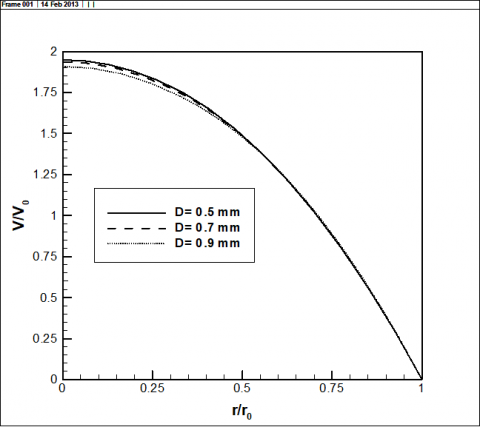Figure 2. Profiles of axial velocity for different tube diameters at z =0.1 and Re= Re= 80

## 3.pngFigure 3. Average Nusselt number versus Reynolds number for different tube diameters

## 4.png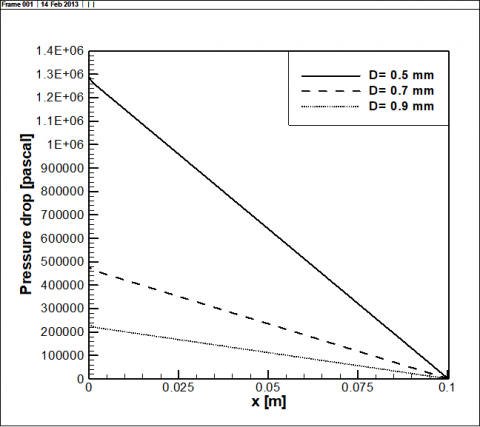Figure 4. Pressure drop along the tube axis for different tube diameters at Re =80

3.2 Effect of entrance size

Three different entrance sizes were investigated in this section. The configuration for different entrance is illustrated in Figure 5. According to the entrance sizes three different diameters which is 0.371 mm, 0.9 mm and 2.5 mm were investigated. The results as shown in Figure 6 show that though the entrance region is just 40% from the tube length but it affects the heat transfer rate through the tube. It is evident that 2.5 mm entrance diameter has the highest Nusselt number followed by 0.9 mm, 0.371 mm respectively. This is because the Nusselt number is proportional directly with the entrance size.

## 5a.png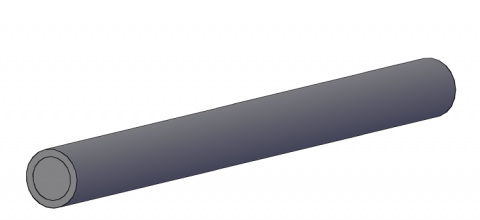(a)

## 5b.png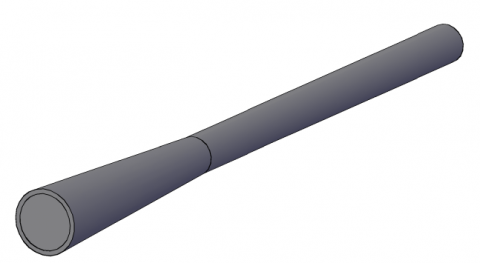(b)

## 5c.png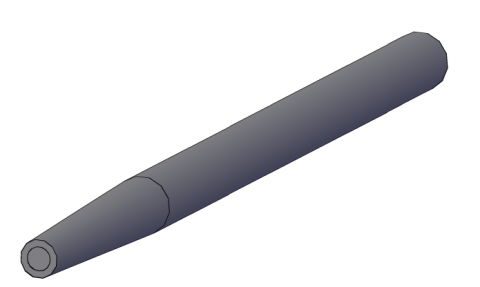(c)

Figure 5. The configuration for different entrance sizes: (a) D= 0.9 mm; (b) D=2.5 mm; (c) D=0.371 mm

## 6.png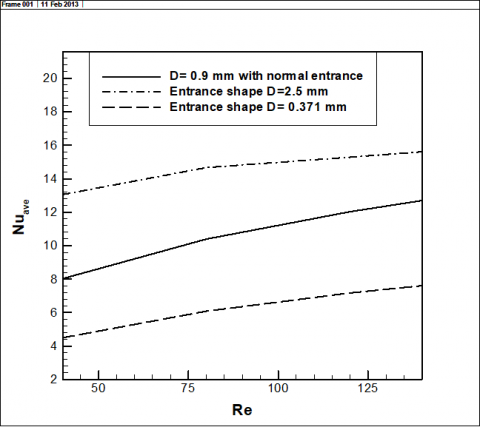Figure 6. Average Nusselt number versus Reynolds number for different entrance sizes

3.3 Effect of inclination angles

Different inclination angles were investigated in this section.  The configuration for different inclination angles is shown in Figure 7. The results for different inclination angles show no changes in the Nusselt number values and the pressure drop at different Reynolds numbers as shown in Table 3. This is because no effect of the gravity, the Richardson number which is a function of Grashof number divided on the Reynolds number is less than Ri ˂ 0.1, so the natural convection is neglected and the forced convection is dominated.

## 7.png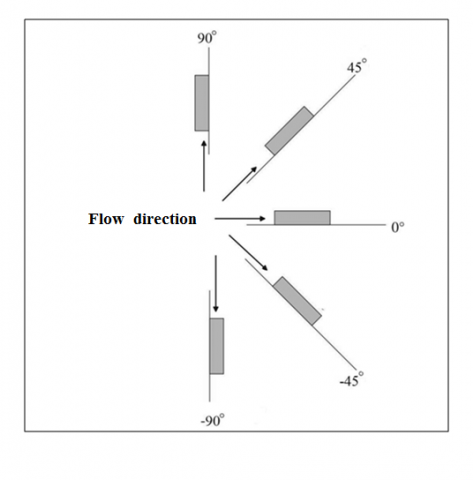Figure 7. Configuration of microtube at different inclination angles

Table 3. The effects of inclination angle on Nusselt number average and pressure drop at different Reynolds number

(a) At Reynolds number 10

 Angle Nuave Pressure 0° 7.362852 79515.71 ± 45° 7.362852 79515.71 ± 90° 7.362852 79515.71

(b) At Reynolds number 40

 Angle Nuave Pressure 0° 10.11316 317295.8 ± 45° 10.11316 317295.8 ± 90° 10.11316 317295.8

(c) At Reynolds number 80

 Angle Nuave Pressure 0° 11.75585 638552.4 ± 45° 11.75585 638552.4 ± 90° 11.75585 638552.4

(d) At Reynolds number 120

 Angle Nuave Pressure 0° 12.86489 960093.9 ± 45° 12.86489 960093.9 ± 90° 12.86489 960093.9
4. Conclusions

The effect of geometrical parameters of microtube on the heat transfer enhancement was investigated numerically. It is concluded from the results that the heat transfer rate strongly depends on the geometry and the entrance size of the microtube. Higher tube diameter and entrance size have the highest Nusselt number and vice versa. No effects of inclination angle on heat transfer rate were found. The buoyancy force is neglected because high values of Reynolds number was used in this investigation. An investigation for the effects of natural convection is needed for the future studies.

Nomenclature
 Al2O3 Aluminum oxide Cp Specific heat of the fluid, J/ kgK CuO Copper oxide df Diameter of base fluid molecule dp Nanoparticle diameter, m g Gravitational acceleration, $m / s^{2}$ k Thermal conductivity, $W / m K$ keff Effective thermal conductivity L Length of the tube, m M Molecular weight of base fluid Avogadro No $N=6.022 \times 10^{23} \mathrm{mol}^{-1}$ Nu Nusselt number, P Pressure of fluid, $P a$ Pr Prandtl number, $P r=C p \mu_{0} / k$ qx Heat flux, $W / m^{2}$ Re Reynolds number, $R e=\rho_{0} V_{0} D / \mu_{0}$ Rnp Nanoparticle radius, m Ri Richardson number, $R i=G r / R e$ SiO2 Silicon oxide T Temperature of fluid, K T Bulk temperature, K T0 Reference temperature U´, V´, W´ Velocities in x´, y´ and z´ directions, $m / s$ U, V, W Dimensionless velocities in x, y and z directions U0 Average jet velocity at the entrance, $m / s$ V Axial velocity, $m / s$ ZnO Zinc oxide Greek symbols μeff Effective viscosity α Thermal diffusivity, $m^{2} / s$ μ Dynamic viscosity of fluid, $\mathrm{kg} / \mathrm{m} \mathrm{s}$ υ Kinematic viscosity of fluid,   $m^{2} s$ θ Inclination of tilted wall ρ Fluid density, $\mathrm{kg} / \mathrm{m}^{3}$ ρ1 Nanofluid density $\mathrm{kg} / \mathrm{m}^{3}$ φ Volume fraction of nanoparticles Subscript bf base-fluid nf nanofluids np nanoparticle eff effective
References

1. S. Arcidiacono, N. R. Bieri, D. Poulikakos and C. P. Grigoropoulos, On the Coalescence of Gold Nanoparticles, International Journal of Multiphase Flow, vol. 30, pp. 979-994, 2004. DOI: 10.1016/j.ijmultiphaseflow.2004.03.006.

2. P. Buffat and J. P. Borel, Size Effect on Melting Temperature of Gold Particles, Physical Review A, vol. 13(6), pp. 2287-2298, 1976. DOI: 10.1103/PhysRevA.13.2287.

3. Zhuo Li, Ya-Ling He, Gui-Hua Tang, Wen-Quan Tao, Experimental and Numerical Studies of Liquid Flow and Heat Transfer in Microtubes, Int. J. Heat and Mass Transfer, vol. 50, pp. 3447–3460, 2007. DOI: 10.1016/j.ijheatmasstransfer.2007.01.016.

4. Renqiang Xiong, J. N. Chung, A New Model for Three- Dimensional Random Roughness Effect on Friction Factor and Heat Transfer in Microtubes, Int. J. Heat and Mass Transfer, vol. 53, pp. 3284–3291, 2010. DOI: 10.1016/j.ijheatmasstransfer.2010.02.050.

5. Sun-Xiao Zhang, Ya-Ling He, Guy Lauriat and Wen- Quan Tao, Numerical Studies of Simultaneously Developing Laminar Flow and Heat Transfer in Microtubes with Thick Wall and Constant Outside Wall Temperature, Int. J. Heat and Mass Transfer, vol. 53, pp. 3977–3989, 2010. DOI: 10.1016/j.ijheatmasstransfer.2010.05.017.

6. Giulio and Paola, Numerical Analysis of Roughness Effect on Microtube Heat Transfer, J. Superlattices and Microstructures, vol. 35, pp. 601–616, 2004. DOI: 10.1016/j.spmi.2003.09.014.

7. Dorin Lelea, Shigefumi Nishio and Kiyoshi Takano, The Experimental Research on Microtube Heat Transfer and Fluid Flow Of Distilled Water, Int. J. Heat and Mass Transfer, vol. 47, pp. 2817–2830, 2004. DOI: 10.1016/j.ijheatmasstransfer.2003.11.034.

8. Yulong Ding, Hajar Alias, Dongsheng Wen and Richard

A. Williams, Heat transfer of aqueous suspensions of carbon nanotubes (CNT nanofluids), Int. J. Heat and Mass Transfer, vol. 49, pp. 240–250, 2006. DOI: 10.1016/j.ijheatmasstransfer.2005.07.009.

9. C. Y. Yang, T. Y. Lin, Heat Transfer Characteristics of Water Flow in Microtubes, Experimental Thermal and Fluid Science, vol. 32, pp. 432–439, 2007. DOI: 10.1016/j.expthermflusci.2007.05.006.

10. R. Kamali, A. R. Binesh, Numerical Investigation of Heat Transfer Enhancement Using Carbon Nanotube- Based Non-Newtonian Nanofluids, Int. Comm. Heat and Mass Transfer, vol. 37, pp. 1153–1157, 2010. DOI: 10.1016/j.icheatmasstransfer.2010.06.001.

11. Chungpyo Hong, Yutaka Asako, Heat Transfer Characteristics of Gaseous Flows in Microtube with Constant Heat Flux, Applied Thermal Engineering, vol. 28, pp. 1375–1385, 2008. DOI: 10.1016/j.applthermaleng.2007.10.006.

12. V. Bianco, F. Chiacchio, O. Manca, S. Nardini, Numerical Investigation of Nanofluids Forced Convection in Circular Tubes, Applied Thermal Engineering, vol. 29, pp. 3632–3642, 2009. DOI: 10.1016/j.applthermaleng.2009.06.019.

13. Z. Y. GUO and X. B, WU. Compressibility effect on the gas flow and heat transfer in a microtube. Int. J. Heat and Mass Transfer, vol. 40, pp. 3251-3254, 1996. DOI: 10.1016/S0017-9310(96)00323-7.

14. S. M. Ghiaasiaan, T. S. Laker, Turbulent Forced Convection in Microtubes, Int. J. Heat and Mass Transfer, vol. 44, pp. 2777-2782, 2001. DOI: 10.1016/S0017-9310(00)00320-3.

15. G. P. Celata, M. Cumo, S. McPhail, G. Zummo, Characterization of Fluid Dynamic Behavior and Channel Wall Effects in Microtube, Int. J. Heat and Mass Transfer, vol. 27, pp. 135–143, 2006. DOI: 10.1016/j.ijheatfluidflow.2005.03.012.

16. Nian Xiao, John Elsnab, Tim Ameel, Microtube Gas Flows with Second-Order Slip Flow and Temperature Jump Boundary Conditions, Int. J. Thermal Sciences, vol. 48, pp. 243–251, 2009. DOI: 10.1016/j.ijthermalsci.2008.08.007.

17.  Peng-Fei Hao, Xi-Wen Zhang, Zhao-Hui Yao, Feng He. Transitional and Turbulent Flow in a Circular Microtube, Experimental Thermal and Fluid Science, vol. 32, pp. 423–431, 2007. DOI: 10.1016/j.expthermflusci.2007.05.004.

18. G. P. Celata, M. Cumo, V. Marconi, S. J. McPhail, G. Zummo, Microtube Liquid Single-Phase Heat Transfer in Laminar Flow, Int. J. Heat and Mass Transfer, vol. 49, pp. 3538–3546, 2006. DOI: 10.1016/j.ijheatmasstransfer.2006.03.004.

19. J. Koo, C. Kleinstreuer, Viscous Dissipation Effects in Microtubes and Microchannels, Int. J. Heat and Mass Transfer, 47:3159–3169, 2004. DOI: 10.1016/j.ijheatmasstransfer.2004.02.017.

20. Gh. Mohiuddin Mala, Dongqing Li, Flow Characteristics of Water in Microtubes, Int. J. Heat and Fluid Flow, vol. 20, pp.142-148, 1999. DOI: 10.1016/S0142-727X(98)10043-7.

21. O. N. Sara, O. Barlay Ergu, M. E. Arzutug, S. Yapici, Experimental Study of Laminar Forced Convective Mass Transfer and Pressure Drop in Microtubes, Int. J. Thermal Sciences, vol. 48, pp. 1894–1900, 2009. DOI: 10.1016/j.ijthermalsci.2009.01.021.

22. Dorin Lelea, Adrian Eugen Cioabla, The Viscous Dissipation Effect on Heat Transfer and Fluid Flow in Micro-Tubes, Int. Comm. Heat and Mass Transfer, vol. 37, pp. 1208–1214, 2010. DOI: 10.1016/j.icheatmasstransfer.2010.06.030.

23. Ashok K. Satapathy, Slip Flow Heat Transfer in an Infinite Microtube with Axial Conduction, Int. J. Thermal Sciences, vol. 49, pp. 153–160, 2010. DOI: 10.1016/j.ijthermalsci.2009.06.012.

24. P. Zhang, X. Fu, Two-Phase Flow Characteristics of Liquid Nitrogen in Vertically Upward 0.5 And1.0 Mm Micro-Tubes: Visualization Studies, Cryogenics, vol. 49, pp. 565–575, 2009. DOI: 10.1016/j.cryogenics.2008.10.017.

25. Haoli Wang, Yuan Wang, Influence of Three- Dimensional Wall Roughness on the Laminar Flow in Microtube, Int. J. Heat and Fluid Flow, vol. 28, pp. 220–228, 2007. DOI: 10.1016/j.ijheatfluidflow.2006.08.005.

26. Zhi-Gang Liu, Shi-Qiang Liang, Masahiro Takei, Experimental Study on Forced Convective Heat Transfer Characteristics in Quartz Microtube, Int. J. Thermal Sciences, vol. 46, pp.139–148, 2007. DOI: 10.1016/j.ijthermalsci.2006.03.008.

27.  G. P. Celata, M. Cumo, S. J. McPhail, L. Tesfagabir, G. Zummo, Experimental Study on Compressible Flow in Microtubes. Int. J. Heat and Fluid Flow, vol. 28, pp. 28–36, 2007. DOI: 10.1016/j.ijheatfluidflow.2006.04.009.

28. A. Aziz, Nick Niedbalski, Thermally Developing Microtube Gas Flow with Axial Conduction and Viscous Dissipation, Int. J. Thermal Sciences, vol. 50, pp. 332–340, 2011. DOI: 10.1016/j.ijthermalsci.2010.08.003.

29. S. L. Qi, P. Zhang, R. Z. Wang, L. X. Xu, Flow Boiling of Liquid Nitrogen in Micro-Tubes: Part II–Heat Transfer Characteristics and Critical Heat Flux, Int. J. Heat and Mass Transfer, vol. 50, pp. 5017–5030, 2007. DOI: 10.1016/j.ijheatmasstransfer.2007.08.017.

30. Dongsheng Wen, Yulong Ding, Experimental Investigation into Convective Heat Transfer of Nanofluids at the Entrance Region under Laminar Flow Conditions, Int. J. Heat and Mass Transfer, vol. 47, pp. 5181–5188, 2004. DOI: 10.1016/j.ijheatmasstransfer.2004.07.012.

31. B. H. Salman, H. A. Mohammed, A. SH. Kherbeet, Heat Transfer Enhancement of Nanofluids Flow in Microtube With Constant Heat Flux, J. Int. Comm. Heat and Mass Transfer, vol. 39, pp. 1195-1204, 2012. DOI: 10.1016/j.icheatmasstransfer.2012.07.005.

32. B. Ghasemi, S. Aminossadati, Brownian Motion of Nanoparticles in a Triangular Enclosure with Natural Convection, J. Thermal Sciences, vol. 49,pp. 931–940, 2010. DOI: 10.1016/j.ijthermalsci.2009.12.017.

33. R. S. Vajjha, D. K. Das, Experimental Determination of Thermal Conductivity of Three Nanoﬂuids and Development of New Correlations, J. Heat and Mass Transfer, vol. 52, pp. 4675–4682, 2009. DOI: 10.1016/j.ijheatmasstransfer.2009.06.027.

34. M. Corcione, Heat Transfer Features of Buoyancy- Driven Nanoﬂuids inside Rectangular Enclosures Differentially Heated at the Sidewalls, J. Thermal Sciences, vol. 49, pp. 1536–1546, 2010. DOI: 10.1016/j.ijthermalsci.2010.05.005.

35. S.V. Patankar, Numerical Heat Transfer and Fluid Flow, Hemisphere, New York, 1980

36. A. A. Minea, Effect of Microtube Length on Heat Transfer Enhancement of a Water/Al2O3 Nanoﬂuid at High Reynolds Numbers, Int. J. Heat and Mass Transfer, vol. 62, pp. 22-30, 2013. DOI: 10.1016/j.ijheatmasstransfer.2013.02.057.

37. B. H. Salman, H. A. Mohammed, K. M. Munisamy, A. Sh. Kherbeet, Characteristics of Heat Transfer and Fluid Flow in Microtube and Microchannel Using Conventional Fluids and Nanofluids: A Review: Renewable and Sustainable Energy Reviews, vol. 28, pp. 848-880, 2013. DOI: 10.1016/j.rser.2013.08.012.

38. B. H. Salman, H. A. Mohammed, K. M. Munisamy, A. Sh. Kherbeet, Three-Dimensional Numerical Investigation of Nanofluids Flow in Microtube with Different Values of Heat Flux, Heat Transfer—Asian Research, 2014. DOI: 10.1002/htj.21139.